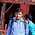ESIC Online Test

ESIC Online Test: ESIC Online Test is available here; Applicants who have applied for ESIC exam must solve online Practice test through this page at free of cost. As online practice test is the best way to Check Your Skill. These questions are taken from prevoius years question papers. By answering these questions, you can check your skills and strenght. Each question has four answers you have to select right one from the four. We provided the answers below the question in detailed format so you can evaluate your performace at same time.

Points to remember:
• Read All Questions Carefully
• Manage Your Time
• Each question carries 1 mark

ESIC Online Test

Study The Following Table And Answer The Questions (1 To 5) Based On It.

Expenditures of a Company (in Lakh Rupees) per Annum Over the given Years.

 Year Item of Expenditure Salary Fuel and Transport Bonus Interest on Loans Taxes 1998 288 98 3.00 23.4 83 1999 342 112 2.52 32.5 108 2000 324 101 3.84 41.6 74 2001 336 133 3.68 36.4 88 2002 420 142 3.96 49.4 98

Question 1 What is the average amount of interest per year which the company had to pay during this period?

A.    Rs. 32.43 lakhs
B.    Rs. 33.72 lakhs
C.    Rs. 34.18 lakhs
D.   Rs. 36.66 lakhs

Question 2 The total amount of bonus paid by the company during the given period is approximately what percent of the total amount of salary paid during this period?

A.    0.1%
B.    0.5%
C.    1%
D.   1.25%

Question 3 Total expenditure on all these items in 1998 was approximately what percent of the total expenditure in 2002?

A.    62%
B.    66%
C.    69%
D.   71%

Question 4 The total expenditure of the company over these items during the year 2000 is?

A.    Rs. 544.44 lakhs
B.    Rs. 501.11 lakhs
C.    Rs. 446.46 lakhs
D.   Rs. 478.87 lakhs

Question 5 The ratio between the total expenditure on Taxes for all the years and the total expenditure on Fuel and Transport for all the years respectively is approximately?

A.    4:7
B.    10:13
C.    15:18
D.   5:8

Question 6 28, 24, 22,18,16,12…

A.    8
B.    12
C.    10
D.   14

 Take a Test  Now

Question 7 50 -14÷4×2 =

A.    43
B.    42
C.    44
D.    46

Question 8 Pointing to shivam, vasu said, "I am the only son of one of the sons of his father." How is shivam related to vasu?

A.    Father or Uncle
B.    Father
C.    Brother
D.   Uncle

Question 9 The small child does whatever his father was done.

A.    Does
B.    Did
C.    has done
E.    No correction required

Question 10 If shivam can complete a work in 10 days and tarun can do the same work in 15 days then how many days they take to complte same work if they work together?

A.    4
B.    6
C.    8
D.   5

Question 11 40, 40, 27,27,14,14…

A.    1
B.    2
C.    3
D.   4

Question 12 Introducing a man priya said, "He is the son of the only son of my grandfather." How is the man in the photograph related to priya?

A.    Data is inadequate
B.    Son
C.    Uncle
D.   Brother

Question 13 Select the odd one out

A.    Canteen
B.    Dancer
C.    Stage
D.   Makeup

Question 14 565, 3396, 23779, ?

A.    1870240
B.    1770140
C.    1678950
D.   1970240

 Check Some Other Important Maths Preparation Links

Question 15 (25+35) ^3 is:

A.    58500
B.    65899
C.    58125
D.   52321
E.    61254

Question 16 1, 2, 3, 4 and 5 are sitting on a bench. 1 is sitting next to 2, 3 is sitting next to 4, 4 is not sitting with 5 who is on the left end of the bench. 3 is on the second position from the right. 1 is to the right of 2 and 5. 1 and 3 are sitting together. In which position 1 is sitting?

A.    Between 2 and 4
B.    Between 2 and 3
C.    Between 5 and 4
D.   Between 3 and 5

Question 17 ELFA, GLHA, ILJA, _____, MLNA

A.    OLPA
B.    KLMA
C.    KLLA
D.   LLMA

Directions (Question Numbers 18-20)

A.    Only Conclusion I follows
B.    Only conclusion II follows
C.    Either conclusion I or II follows
D.   Neither conclusion I or II follows
E.    Both conclusions I and II follow

Question 18 Statements

Zinat>Tanmay, Tanmay<Mayank, Mayank<Jeevan

Conclusions
A.    Tanmay<Jeevan
B.    Jeevan<Zinat

Question 19 Statements
Queen=Zebra, Cat>=Got, Got>=Queen, Queen>=Rat, Joy>=Cat

Conclusions
A.    Gagan>=Zinu
B.    Cat>=Rat

 Take Help From These Preparation Stuff

Question 20 Statements

Animal>Buffalo>Cow, Duck>Eagle>Fox, Duck>Cow

Conclusions
A.    Eagle>Cow
B.    Fox>Buffalo

1.